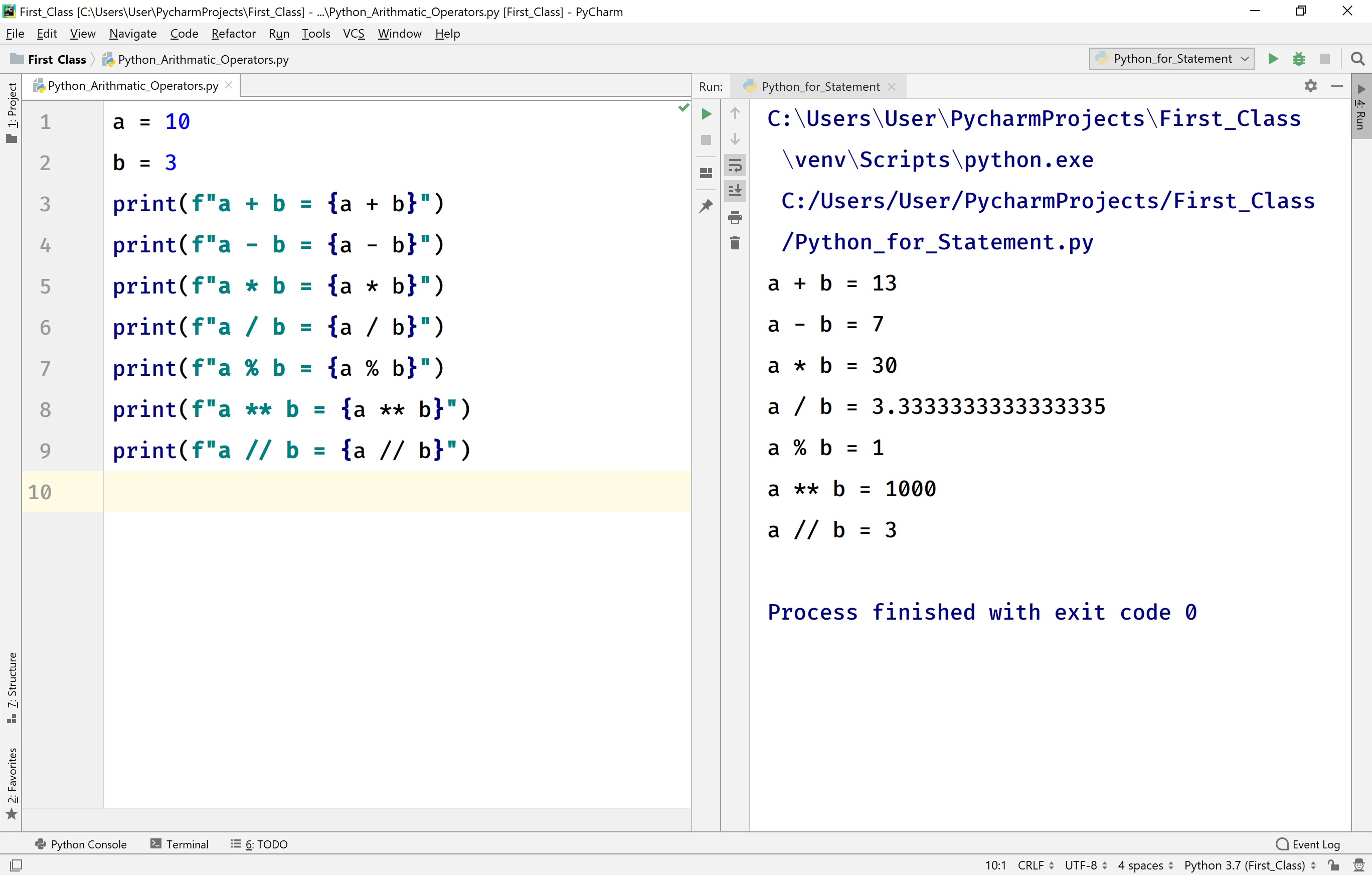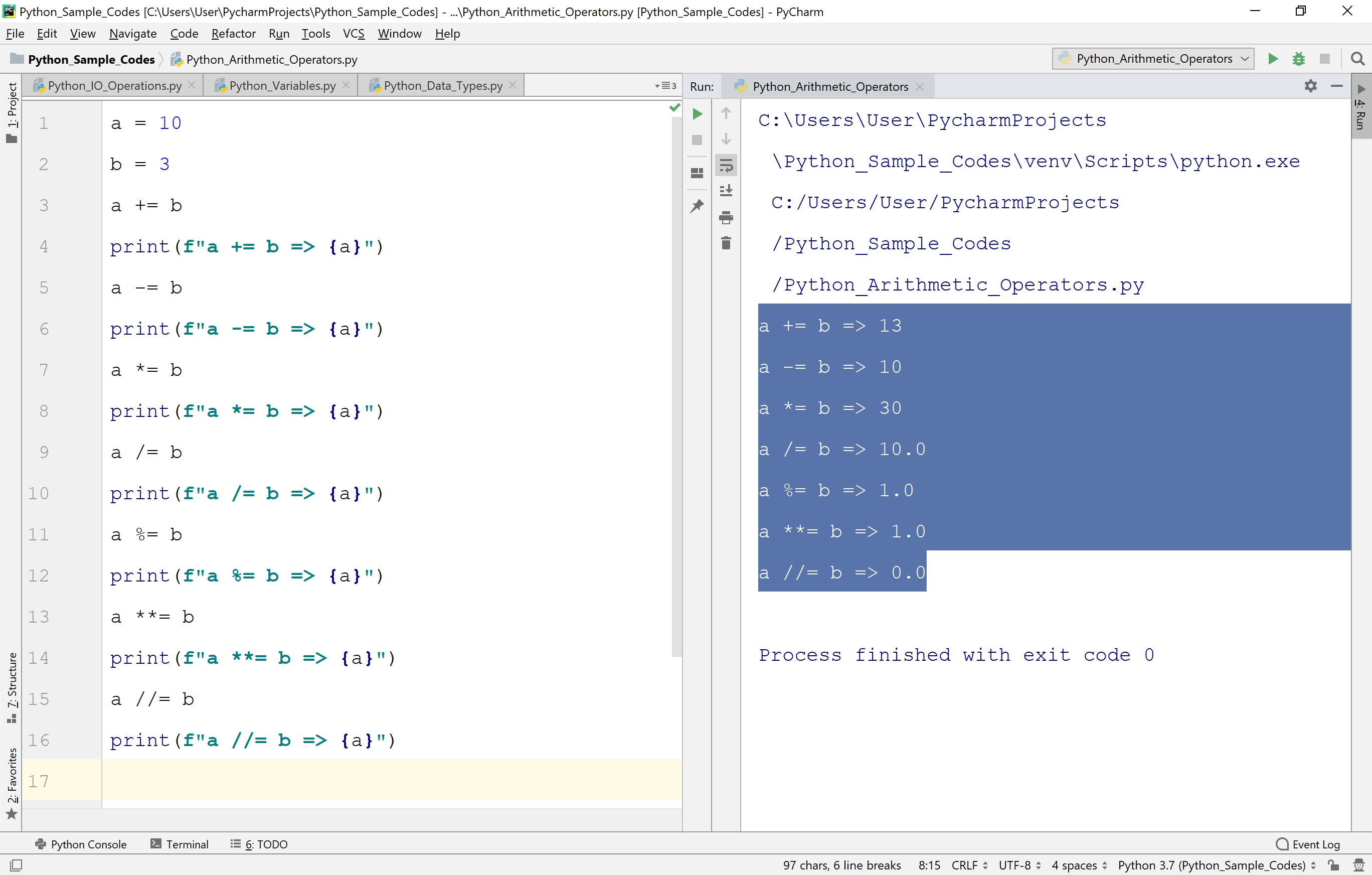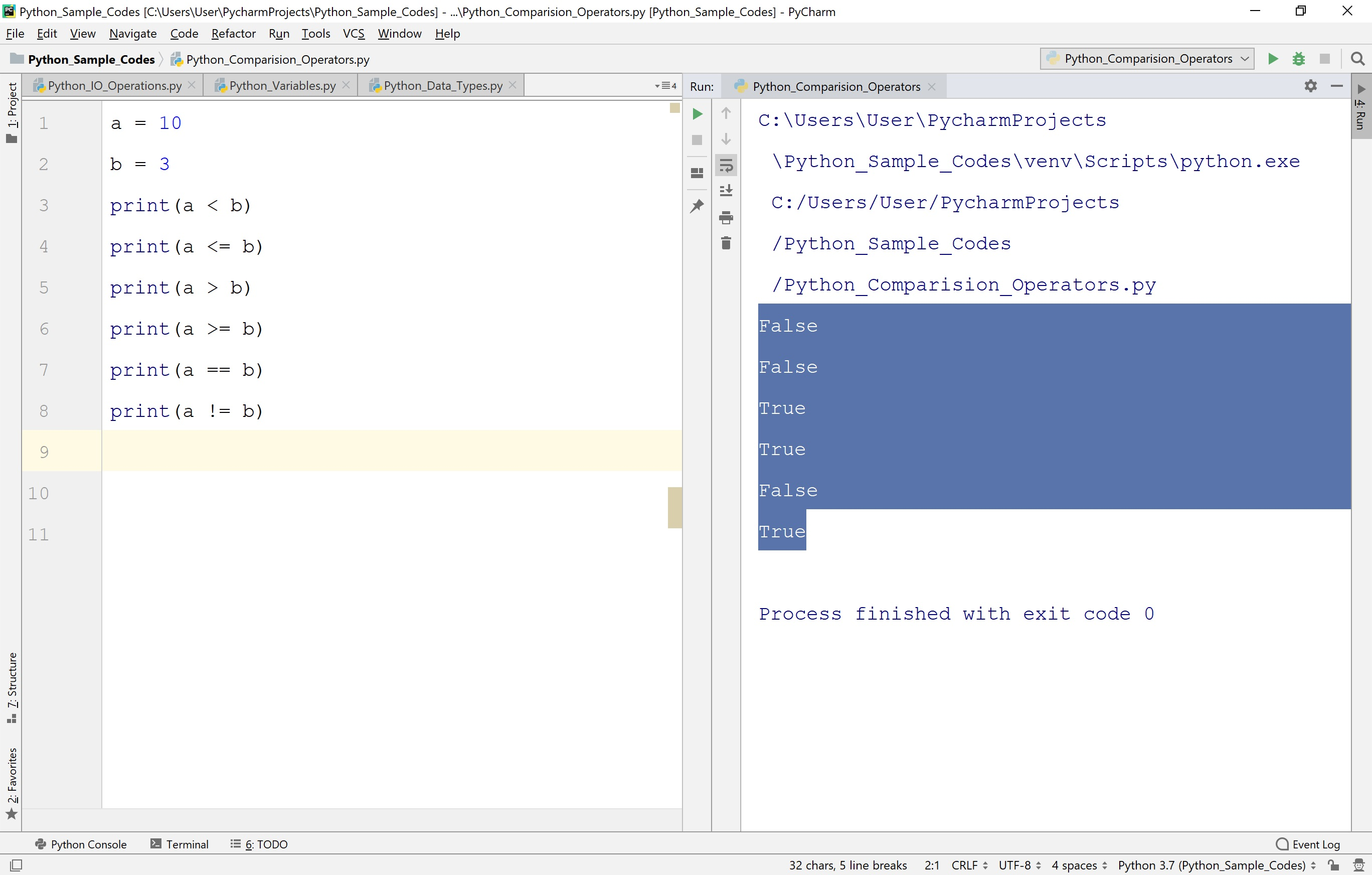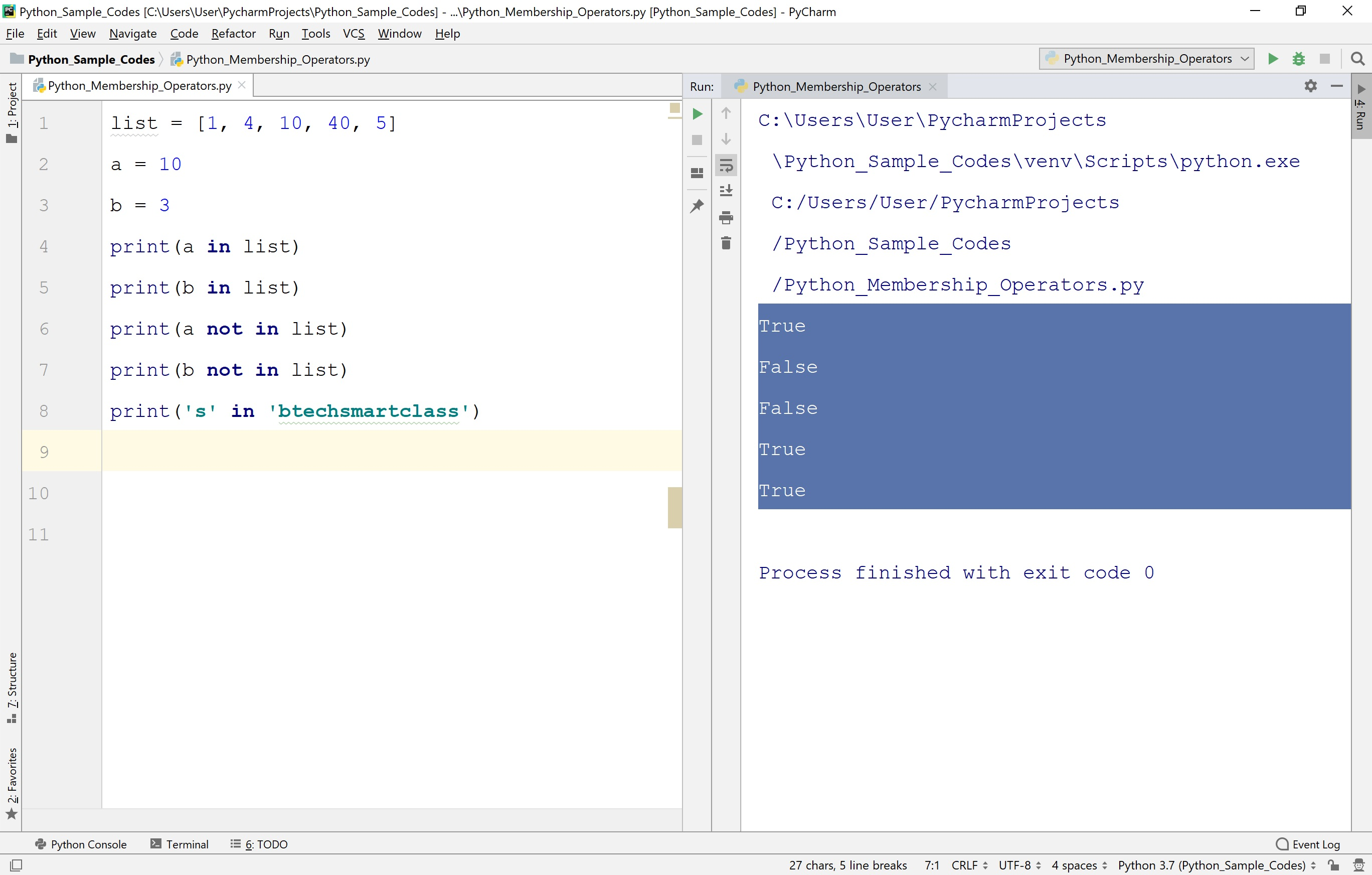The perfect place for easy learning...

×

# Python Operators

In Python, an operator is a symbol used to perform arithmetical and logical operations. In other words, an operator can be defined as a symbol used to manipulate the value of an operand. Here, an operand is a value or variable on which the operator performs its task. For example, '+' is a symbol used to perform mathematical addition operation.

Consider the expression a = 10 + 30 . Here, variable 'a', values '10' and '30' are known as Operands and the symbols '=' and '+' are known as Operators.

## Types of Operators in Python

In Python, there is a rich set of operators, and they are classified as follows.

• Arithmetic Operators ( +, -, *, /, %, **, // )
• Assignment Operators ( =, +=, -=, *=, /=, %=, **=, //= )
• Comparison Operators ( <, <=, >, >=, ==, != )
• Logical Operators ( and, or, not )
• Identity Operators ( is, is not )
• Membership Operators ( in, not in )
• Bitwise Operators ( &, |, ^, ~, <<, >> )

Let's look at each type of operators in Python.

## Arithmetic Operators in Python

In Python, the arithmetic operators are the operators used to perform a basic arithmetic operation between two variables or two values. The following table presents the list of arithmetic operations in Python along with their description.

To understand the example let's consider two variables a with value 10 and b with value 3.

Operator Meaning Description Example
+ Addition Performs mathematical addition on both side of the operator a + b = 13
- Subtraction Subtracts the right-hand operand from the left-hand operand a - b = 7
* Multiplication Multiplies values on both sides of the operator a * b = 30
/ Division Performs division by Dividing left-hand operand by right-hand operand a / b = 3.3333333333333335
% Modulus Performs division by Dividing left-hand operand by right-hand operand and returns remainder a % b = 1
** Exponent Performs exponential (power) calculation on operators a ** b = 1000
// Floor Division Performs division which the digits after the decimal point are removed. But if one of the operands is negative, the result is rounded away from zero (towards negative infinity) a // b = 3

Let's look at the following example code to illustration the arithmetic operators in Python.

# Example - Arithmetic Operators in Python

``````
a = 10
b = 3
print(f"a + b = {a + b}")
print(f"a - b = {a - b}")
print(f"a * b = {a * b}")
print(f"a / b = {a / b}")
print(f"a % b = {a % b}")
print(f"a ** b = {a ** b}")
print(f"a // b = {a // b}")
```
```

When we run the above code, it produces the output as follows.## Assignment Operators in Python

In Python, the assignment operators are the operators used to assign the right-hand side value to the left-hand side variable. The following table presents the list of assignment operations in Python along with their description.

To understand the example let's consider two variables a with value 10 and b with value 3.

Operator Meaning Description Example
= Assignment Assigns right-hand side value to left-hand side variable a = 10
+= Add and Assign Adds right operand to the left operand and assign the result to left operand a += b
≈ ( a = a + b )
-= Subtract and Assign Subtracts right operand from the left operand and assign the result to left operand a -= b
≈ ( a = a - b )
*= Multiply and Assign Multiplies right operand with the left operand and assign the result to left operand a *= b
≈ ( a = a * b )
/= Division and Assign Divides left operand with the right operand and assign the result to left operand a /= b
≈ ( a = a / b )
%= Modulus and Assign Takes modulus using two operands and assign the result to left operand a %= b
≈ ( a = a % b )
**= Exponent and Assign Performs exponential calculation on operators and assigns value to the left operand a **= b
≈ ( a = a ** b )
//= Floor Division and Assign Performs floor division on operators and assign value to the left operand a //= b
≈ ( a = a // b )

Let's look at the following example code to illustration the assignment operators in Python.

# Example - Assignment Operators in Python

``````
a = 10
b = 3
a += b
print(f"a += b => {a}")
a -= b
print(f"a -= b => {a}")
a *= b
print(f"a *= b => {a}")
a /= b
print(f"a /= b => {a}")
a %= b
print(f"a %= b => {a}")
a **= b
print(f"a **= b => {a}")
a //= b
print(f"a //= b => {a}")
```
```

When we run the above code, it produces the output as follows.## Comparison Operators in Python

In Python, the comparison operators are used to compare two values. In other words, comparison operators are used to checking the relationship between two variables or values. The comparison operators are also known as Relational Operators. The following table presents the list of comparison operations in Python along with their description.

To understand the example let's consider two variables a with value 10 and b with value 3.

Operator Meaning Description Example
< Less than Returns True if left-hand side value is smaller than right-hand side value, otherwise returns False. a < b
(False)
<= Less than or Equal to Returns True if left-hand side value is smaller than or equal to right-hand side value, otherwise returns False. a <= b
( False )
> Greater than Returns True if left-hand side value is larger than right-hand side value, otherwise returns False. a > b
( True )
>= Graeter than or Equal to Returns True if left-hand side value is larger than or equal to right-hand side value, otherwise returns False. a >= b
( True )
== Equal to Returns True if left-hand side value is equal to right-hand side value, otherwise returns False. a == b
( False )
!= Not equal to Returns True if left-hand side value is not equal to right-hand side value, otherwise returns False. a != b
( True )

Let's look at the following example code to illustration the comparison operators in Python.

# Example - Comparison Operators in Python

``````
a = 10
b = 3
print(a < b)
print(a <= b)
print(a > b)
print(a >= b)
print(a == b)
print(a != b)
```
```

When we run the above code, it produces the output as follows.## Logical Operators in Python

In Python, the logical operators are used to merge multiple conditions into a single condition. The following table presents the list of logical operations in Python along with their description.

To understand the example let's consider two variables a with value 10 and b with value 3.

Operator Meaning Description Example
and Logical AND Returns True if all the conditions are True, otherwise returns False. a < b and a > c
or Logical OR Returns False if all the conditions are False, otherwise returns True. a < b or a > c
not Logical NOT Returns True if the condition is False, otherwise returns False. not a > b

Let's look at the following example code to illustration the logical operators in Python.

# Example - Logical Operators in Python

``````
a = 10
b = 3
c = 20
print(a < b and a > c)
print(a < b or a > c)
print(not a > b)
```
```

When we run the above code, it produces the output as follows.## Identity Operators in Python

In Python, identity operators are used to comparing the memory locations of two objects or variables. There are two identity operators in Python. The following table presents the list of identity operations in Python along with their description.

To understand the example let's consider two variables a with value 10 and b with value 3.

Operator Meaning Description Example
is is identical Returns True if the variables on either side of the operator point to the same object otherwise returns False. a is b
is not is not identical Returns False if the variables on either side of the operator point to the same object otherwise returns True. a is not b

Let's look at the following example code to illustration the identity operators in Python.

# Example - Identity Operators in Python

``````
a = 10
b = 3
print(a is b)
print(a is not b)
```
```

When we run the above code, it produces the output as follows.## Membership Operators in Python

In Python, the membership operators are used to test whether a value is present in a sequence. Here the sequence may be String, List, or Tuple. There are two membership operators in Python. The following table presents the list of membership operations in Python along with their description.

To understand the example let's consider two variables a with value 10, b with value 3, and a list [1, 4, 10, 40, 5].

Operator Meaning Description Example
in in Returns True if it finds a variable in the specified sequence, otherwise returns False. a in list
not in not in Returns True if it does not finds a variable in the specified sequence, otherwise returns False. a not in list

Let's look at the following example code to illustration the membership operators in Python.

# Example - Membership Operators in Python

``````
list = [1, 4, 10, 40, 5]
a = 10
b = 3
print(a in list)
print(b in list)
print(a not in list)
print(b not in list)
print('s' in 'btechsmartclass')
```
```

When we run the above code, it produces the output as follows.## Bitwise Operators in Python

In Python, the bitwise operators are used to performs bit by bit operation. There are six bitwise operators in Python. The following table presents the list of bitwise operations in Python along with their description.

To understand the example let's consider two variables a with value 10 equivalent binary value is 1010, b with value 15 equivalent binary value is 1111.

Operator Meaning Description Example
& Bitwise AND Returns True if it finds a variable in the specified sequence, otherwise returns False. a in list
| Bitwise OR Returns True if it does not find a variable in the specified sequence, otherwise returns False. a not in list
^ Bitwise Exclusive OR Returns True if it does not find a variable in the specified sequence, otherwise returns False. a not in list
~ Bitwise Ones Complement Returns True if it does not finds a variable in the specified sequence, otherwise returns False. a not in list
<< Bitwise Left Shift Returns True if it does not finds a variable in the specified sequence, otherwise returns False. a not in list
>> Bitwise Right Shift Returns True if it does not finds a variable in the specified sequence, otherwise returns False. a not in list

Let's look at the following example code to illustration the bitwise operators in Python.

# Example - bitwise Operators in Python

``````
a = 10
b = 15
print(f"{bin(a)} & {bin(b)} = {bin(a & b)}")
print(f"{bin(a)} | {bin(b)} = {bin(a | b)}")
print(f"{bin(a)} ^ {bin(b)} = {bin(a ^ b)}")
print(f"~{bin(a)} = {bin(~a)}")
print(f"{bin(a)} << 2 = {bin(a << 2)}")
print(f"{bin(a)} >> 2 = {bin(a >> 2)}")
```
```

When we run the above code, it produces the output as follows.